• ## 手写数字识别

千人学习 2017-06-28 20:34:23
手写数字识别，使用简化后的近邻算法实现手写数字识别c++小游戏 c++游戏 c++链表
• 本文使用Tensorflow框架进行Python编程实现基于卷积神经网络的手写数字识别算法，并将其封装在一个GUI界面中，最终，设计并实现了一个手写数字识别系统。
手写数字识别算法的设计与实现
本文使用python基于TensorFlow设计手写数字识别算法，并编程实现GUI界面，构建手写数字识别系统。这是本人的本科毕业论文课题，当然，这个也是机器学习的基本问题。本博文不会以论文的形式展现，而是以编程实战完成机器学习项目的角度去描述。
项目要求：本文主要解决的问题是手写数字识别，最终要完成一个识别系统。
设计识别率高的算法，实现快速识别的系统。
1 LeNet-5模型的介绍
本文实现手写数字识别，使用的是卷积神经网络，建模思想来自LeNet-5，如下图所示：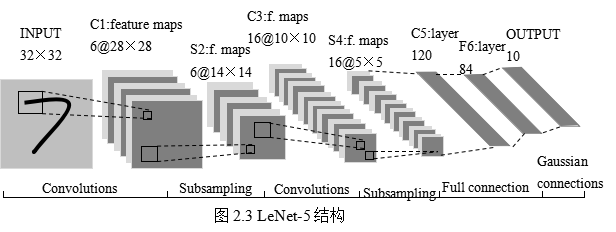这是原始的应用于手写数字识别的网络，我认为这也是最简单的深度网络。
LeNet-5不包括输入，一共7层，较低层由卷积层和最大池化层交替构成，更高层则是全连接和高斯连接。
LeNet-5的输入与BP神经网路的不一样。这里假设图像是黑白的，那么LeNet-5的输入是一个32*32的二维矩阵。同时，输入与下一层并不是全连接的，而是进行稀疏连接。本层每个神经元的输入来自于前一层神经元的局部区域(5×5)，卷积核对原始图像卷积的结果加上相应的阈值，得出的结果再经过激活函数处理，输出即形成卷积层（C层）。卷积层中的每个特征映射都各自共享权重和阈值，这样能大大减少训练开销。降采样层（S层）为减少数据量同时保存有用信息，进行亚抽样。
第一个卷积层（C1层）由6个特征映射构成，每个特征映射是一个28×28的神经元阵列，其中每个神经元负责从5×5的区域通过卷积滤波器提取局部特征。一般情况下，滤波器数量越多，就会得出越多的特征映射，反映越多的原始图像的特征。本层训练参数共6×(5×5+1)=156个，每个像素点都是由上层5×5=25个像素点和1个阈值连接计算所得，共28×28×156=122304个连接。
S2层是对应上述6个特征映射的降采样层（pooling层）。pooling层的实现方法有两种，分别是max-pooling和mean-pooling，LeNet-5采用的是mean-pooling，即取n×n区域内像素的均值。C1通过2×2的窗口区域像素求均值再加上本层的阈值，然后经过激活函数的处理，得到S2层。pooling的实现，在保存图片信息的基础上，减少了权重参数，降低了计算成本，还能控制过拟合。本层学习参数共有1*6+6=12个，S2中的每个像素都与C1层中的2×2个像素和1个阈值相连，共6×(2×2+1)×14×14=5880个连接。
S2层和C3层的连接比较复杂。C3卷积层是由16个大小为10×10的特征映射组成的，当中的每个特征映射与S2层的若干个特征映射的局部感受野（大小为5×5）相连。其中，前6个特征映射与S2层连续3个特征映射相连，后面接着的6个映射与S2层的连续的4个特征映射相连，然后的3个特征映射与S2层不连续的4个特征映射相连，最后一个映射与S2层的所有特征映射相连。此处卷积核大小为5×5，所以学习参数共有6×(3×5×5+1)+9×(4×5×5+1)+1×(6×5×5+1)=1516个参数。而图像大小为28×28，因此共有151600个连接。
S4层是对C3层进行的降采样，与S2同理，学习参数有16×1+16=32个，同时共有16×(2×2+1)×5×5=2000个连接。
C5层是由120个大小为1×1的特征映射组成的卷积层，而且S4层与C5层是全连接的，因此学习参数总个数为120×(16×25+1)=48120个。
F6是与C5全连接的84个神经元，所以共有84×(120+1)=10164个学习参数。
卷积神经网络通过通过稀疏连接和共享权重和阈值，大大减少了计算的开销，同时，pooling的实现，一定程度上减少了过拟合问题的出现，非常适合用于图像的处理和识别。
2 手写数字识别算法模型的构建
2.1 各层设计
有了第一节的基础知识，在这基础上，进行完善和改进。

输入层设计

输入为28×28的矩阵，而不是向量。

激活函数的选取

Sigmoid函数具有光滑性、鲁棒性和其导数可用自身表示的优点，但其运算涉及指数运算，反向传播求误差梯度时，求导又涉及乘除运算，计算量相对较大。同时，针对本文构建的含有两层卷积层和降采样层，由于sgmoid函数自身的特性，在反向传播时，很容易出现梯度消失的情况，从而难以完成网络的训练。因此，本文设计的网络使用ReLU函数作为激活函数。
ReLU的表达式：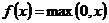卷积层设计

本文设计卷积神经网络采取的是离散卷积，卷积步长为1，即水平和垂直方向每次运算完，移动一个像素。卷积核大小为5×5。

降采样层

本文降采样层的pooling方式是max-pooling，大小为2×2。

输出层设计

输出层设置为10个神经网络节点。数字0~9的目标向量如下表所示：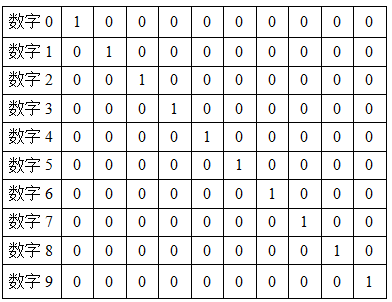2.2 网络模型的总体结构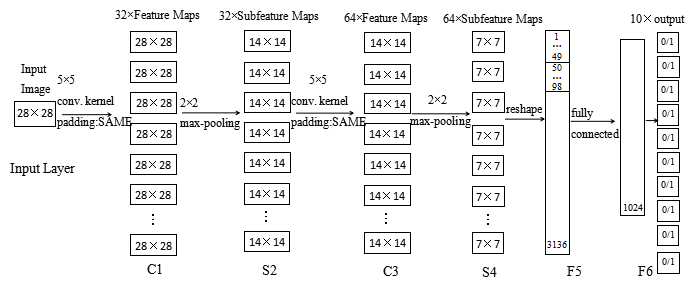其实，本文网络的构建，参考自TensorFlow的手写数字识别的官方教程的，读者有兴趣也可以详细阅读。
2.3 编程实现算法
本文使用Python，调用TensorFlow的api完成手写数字识别的算法。
注：本文程序运行环境是：Win10,python3.5.2。当然，也可以在Linux下运行，由于TensorFlow对py2和py3兼容得比较好，在Linux下可以在python2.7中运行。
#!/usr/bin/env python2
# -*- coding: utf-8 -*-
"""
Created on Fri Feb 17 19:50:49 2017

@author: Yonghao Huang
"""

#import modules
import numpy as np
import matplotlib.pyplot as plt
import tensorflow as tf
import time
from datetime import timedelta
import math
from tensorflow.examples.tutorials.mnist import input_data

def new_weights(shape):
return tf.Variable(tf.truncated_normal(shape,stddev=0.05))
def new_biases(length):
return tf.Variable(tf.constant(0.1,shape=length))
def conv2d(x,W):
def max_pool_2x2(inputx):

#import data
data = input_data.read_data_sets("./data", one_hot=True)  # one_hot means [0 0 1 0 0 0 0 0 0 0] stands for 2

print("Size of:")
print("--Training-set:\t\t{}".format(len(data.train.labels)))
print("--Testing-set:\t\t{}".format(len(data.test.labels)))
print("--Validation-set:\t\t{}".format(len(data.validation.labels)))
data.test.cls = np.argmax(data.test.labels,axis=1)   # show the real test labels:  [7 2 1 ..., 4 5 6], 10000values

x = tf.placeholder("float",shape=[None,784],name='x')
x_image = tf.reshape(x,[-1,28,28,1])

y_true = tf.placeholder("float",shape=[None,10],name='y_true')
y_true_cls = tf.argmax(y_true,dimension=1)
# Conv 1
layer_conv1 = {"weights":new_weights([5,5,1,32]),
"biases":new_biases()}
h_conv1 = tf.nn.relu(conv2d(x_image,layer_conv1["weights"])+layer_conv1["biases"])
h_pool1 = max_pool_2x2(h_conv1)
# Conv 2
layer_conv2 = {"weights":new_weights([5,5,32,64]),
"biases":new_biases()}
h_conv2 = tf.nn.relu(conv2d(h_pool1,layer_conv2["weights"])+layer_conv2["biases"])
h_pool2 = max_pool_2x2(h_conv2)
# Full-connected layer 1
fc1_layer = {"weights":new_weights([7*7*64,1024]),
"biases":new_biases()}
h_pool2_flat = tf.reshape(h_pool2,[-1,7*7*64])
h_fc1 = tf.nn.relu(tf.matmul(h_pool2_flat,fc1_layer["weights"])+fc1_layer["biases"])
# Droupout Layer
keep_prob = tf.placeholder("float")
h_fc1_drop = tf.nn.dropout(h_fc1,keep_prob)
# Full-connected layer 2
fc2_layer = {"weights":new_weights([1024,10]),
"biases":new_weights()}
# Predicted class
y_pred = tf.nn.softmax(tf.matmul(h_fc1_drop,fc2_layer["weights"])+fc2_layer["biases"])  # The output is like [0 0 1 0 0 0 0 0 0 0]
y_pred_cls = tf.argmax(y_pred,dimension=1)  # Show the real predict number like '2'
# cost function to be optimized
cross_entropy = -tf.reduce_mean(y_true*tf.log(y_pred))
# Performance Measures
correct_prediction = tf.equal(y_pred_cls,y_true_cls)
accuracy = tf.reduce_mean(tf.cast(correct_prediction,"float"))
with tf.Session() as sess:
init = tf.global_variables_initializer()
sess.run(init)
train_batch_size = 50
def optimize(num_iterations):
total_iterations=0
start_time = time.time()
for i in range(total_iterations,total_iterations+num_iterations):
x_batch,y_true_batch = data.train.next_batch(train_batch_size)
feed_dict_train_op = {x:x_batch,y_true:y_true_batch,keep_prob:0.5}
feed_dict_train = {x:x_batch,y_true:y_true_batch,keep_prob:1.0}
sess.run(optimizer,feed_dict=feed_dict_train_op)
# Print status every 100 iterations.
if i%100==0:
# Calculate the accuracy on the training-set.
acc = sess.run(accuracy,feed_dict=feed_dict_train)
# Message for printing.
msg = "Optimization Iteration:{0:>6}, Training Accuracy: {1:>6.1%}"
# Print it.
print(msg.format(i+1,acc))
# Update the total number of iterations performed
total_iterations += num_iterations
# Ending time
end_time = time.time()
# Difference between start and end_times.
time_dif = end_time-start_time
# Print the time-usage
print("Time usage:"+str(timedelta(seconds=int(round(time_dif)))))
test_batch_size = 256
def print_test_accuracy():
# Number of images in the test-set.
num_test = len(data.test.images)
cls_pred = np.zeros(shape=num_test,dtype=np.int)
i = 0
while i < num_test:
# The ending index for the next batch is denoted j.
j = min(i+test_batch_size,num_test)
# Get the images from the test-set between index i and j
images = data.test.images[i:j, :]
# Get the associated labels
labels = data.test.labels[i:j, :]
# Create a feed-dict with these images and labels.
feed_dict={x:images,y_true:labels,keep_prob:1.0}
# Calculate the predicted class using Tensorflow.
cls_pred[i:j] = sess.run(y_pred_cls,feed_dict=feed_dict)
# Set the start-index for the next batch to the
# end-index of the current batch
i = j
cls_true = data.test.cls
correct = (cls_true==cls_pred)
correct_sum = correct.sum()
acc = float(correct_sum) / num_test
# Print the accuracy
msg = "Accuracy on Test-Set: {0:.1%} ({1}/{2})"
print(msg.format(acc,correct_sum,num_test))
# Performance after 10000 optimization iterations


运行结果显示：测试集中准确率大概为99.2%。
我还写了一些辅助函数，可以查看部分识别错误的图片，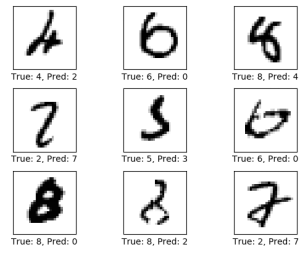还可以查看混淆矩阵，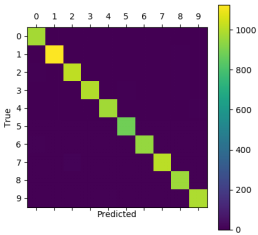2.3 实现手写识别系统
最后，将训练好的参数保存，封装进一个GUI界面中，形成一个手写识别系统。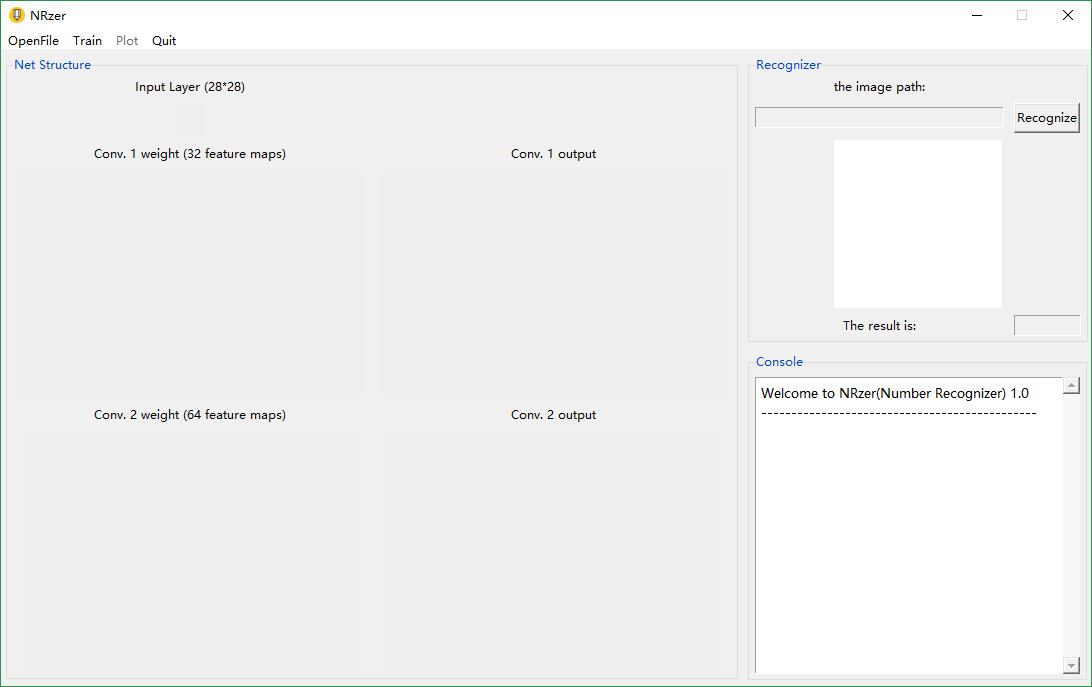系统中还添加了一点图像预处理的操作，比如灰度化，图像信息的归一化等，更贴近实际应用。
系统可进行快速识别，如下图：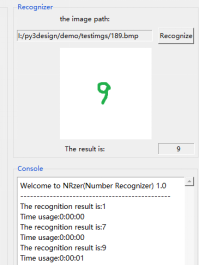3 总结
本文实现的系统其实是基于卷积神经网络的手写数字识别系统。该系统能快速实现手写数字识别，成功识别率高。缺点：只能正确识别单个数字，图像预处理还不够，没有进行图像分割，读者也可以自行添加，进行完善。
4 收获
本人之前的本科期间，虽然努力学习高数、线性代数和概率论，但是没有认真学习过机器学习，本人是2017年才开始系统学习机器学习相关知识，而且本科毕业论文也选择了相关的课题，虽然比较基础，但是认真完成后，有一种学以致用的满足感，同时也激励着我进行更深入的理论学习和实践探讨，与所有读者共勉。
==================================
2018年5月13日更新
以上是基本网络的设计与基本的实现，可满足入门学习。
相关链接:
========================================
2018年6月6日更新更新！！
python(TensorFlow)实现手写字符识别
此处的“手写字符”，其实指的是notMNIST数据库中的手写字符，其实和MNIST数据库是一样的。这里实现手写字符识别，主要是展示TensorFlow框架的可拓展性很强，具体来说，就是可以通过改动少部分的代码，从而实现一个新的识别功能。
NotMnist数据库
这个数据库和MNIST数据库基本一样，只是把10个数字换成了10个字母，即：A,B,C,D,E,F,G,H,I,J,K
当然，这个数据库的识别难度大一些，因为数据噪声更多一些，详情读者可以搜一搜了解一下。
实战
将NotMNIST数据库下载以后，放在本博文上述的网络中，基本不需要修改代码，直接训练，即可得到一个能识别字符的网络模型。
最后在测试集中的准确率，比MNIST的会低一些，大概为96%左右。
本文也将训练好的网络模型封装在和上述系统相似的GUI系统中，
[外链图片转存失败(img-k7xPyAio-1564543116627)(https://i.imgur.com/59M3NlD.png)]
识别效果还可以！
同样，将卷积卷积层可视化。
[外链图片转存失败(img-tIWWgZB9-1564543116629)(https://i.imgur.com/4awe7NY.png)]
结语
TensorFlow框架可拓展性很强，只要设计好了网络，就能很容易的实现出来；同时，使用基本的CNN识别整体架构也是大同小异的，很多识别任务是通用的。当然，在具体的实践中需要得到接近完美的效果，还是要下很大功夫的！努力学习吧，加油！
（如果你/您有什么有趣的想法，可以在下面留言，如果我也感兴趣同时又有时间的话，我会尝试做一做，_）


展开全文机器学习 python Tensorflow
• 手写数字识别程序 手写数字识别程序 手写数字识别程序 手写数字识别程序 手写数字识别程序 手写数字识别程序 手写数字识别程序 手写数字识别程序 手写数字识别程序
•python
• 手写数字的识别。基于人工神经网络的MATLAB手写数字识别系统matlab
• 手写数字的识别。基于人工神经网络的MATLAB手写数字识别系统matlabmatlab
• 手写数字识别问题简介问题描述：手写数字识别是指给定一系列的手写数字图片以及对应的数字标签，构建模型进行学习，目标是对于一张新的手写数字图片能够自动识别出对应的数字。这里主要涉及到的技术是图像识别，图像...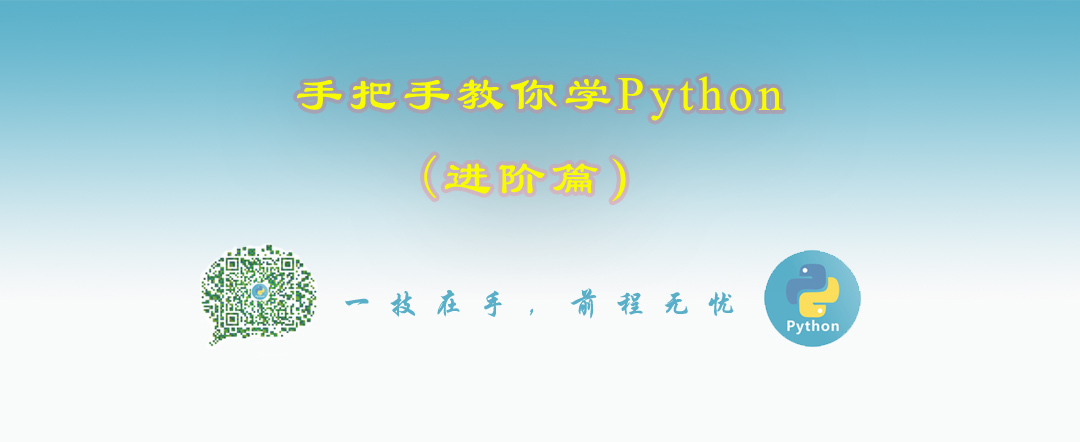手写数字识别问题简介问题描述：手写数字识别是指给定一系列的手写数字图片以及对应的数字标签，构建模型进行学习，目标是对于一张新的手写数字图片能够自动识别出对应的数字。这里主要涉及到的技术是图像识别，图像识别是指利用计算机对图像进行处理、分析和理解，以识别各种不同模式的目标和对像的技术。机器学习领域一般将此类识别问题转化为分类问题。手写数字识别可以转化为10分类问题，最终结果只能是0-9这10个数字。网上手写数字数据集很多，大小也存在差异。这里使用Scikit-learn库中自带的手写数字数据集，其中包含1797个手写数字样本，每个样本是一张8*8的图片，用包含64个元素的一维数组表示，数组元素为0-16之间的整数，表示颜色信息。每个样本都有对应的标签，标签为0-9之间的整数。获取手写数字数据集并显示前36个样本图片的关键代码如下：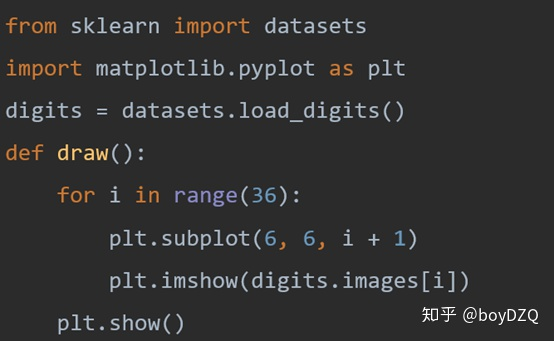数据集中前36个样本显示效果如图所示。由于图片比较小，所以有些马赛克的效果。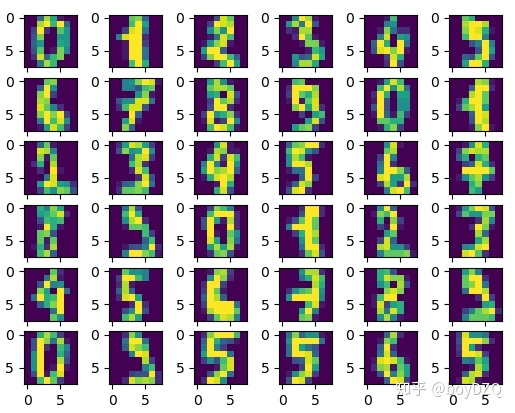多层感知机多层感知机（人工神经网络）是指含有至少一个隐藏层的由全连接层组成的神经网络，其中每一个隐藏层的输出都会通过激活函数进行变换。多层感知机的层数、隐藏层的大小、激活函数都是超参数，可以自己设定。简单的多层感知机示意图如下，包含输入层、隐藏层（可以有多个隐藏层）、输出层。输入层和隐藏层之间、隐藏层与输出层之间都是全连接，每个连接都有相应的权重。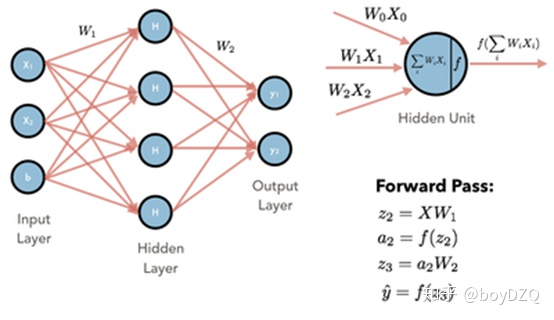参数：各个层之间的连接权重以及偏置；目标：使得误差最小，是一个最优化问题；方法：梯度下降法等；过程：首先随机初始化所有参数，然后迭代地训练，不断地计算梯度和更新参数，直到满足某个条件为止（比如误差足够小、迭代次数足够多）。这个过程涉及到代价函数、规则化、学习速率、梯度计算等。为什么需要激活函数？ 如果不用激活函数，无论神经网络有多少层，输出都是输入的线性组合，这样网络的逼近能力十分有限。引入非线性函数作为激励函数，这样深层神经网络表达能力更强，几乎可以逼近任意函数。常见的激活函数主要有以下几种：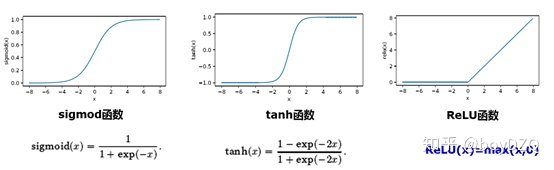使用多层感知机分类器进行手写数字识别的关键代码如下：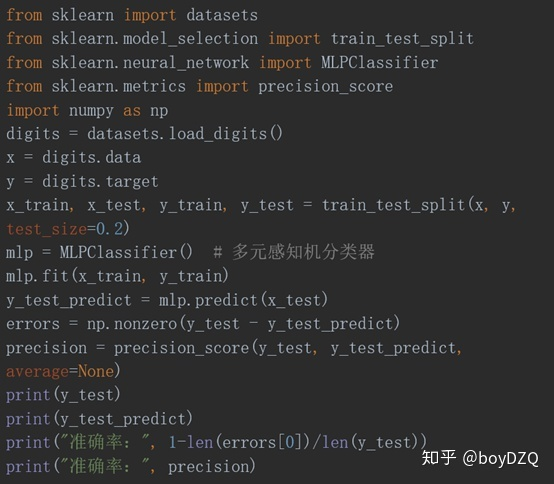MLPClassifier默认隐藏层神经元为100个，激活函数为relu。可改变这两个参数（hidden_layer_sizes、activation），观察执行结果。更多详细内容可查看视频讲解，手把手教你学Python（进阶篇）课程完整视频腾讯课堂链接：https://ke.qq.com/course/2026380?tuin=4c5cc1bc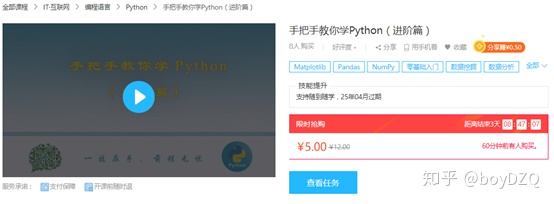更多Python学习资料请加入群、联系助理老师或关注微信公众号获取，我们会不定期更新！展开全文• 文章目录前言手写数字识别神经网络设计数据集使用神经网络设计数学模型关于矩阵求导程序实现运行结果关于权值初始化不同初始化方法收敛速度分析：为什么不同的初始化收敛速度不一样？关于损失函数关于我的感想附录...
文章目录前言手写数字识别神经网络设计数据集使用神经网络设计数学模型关于矩阵求导程序实现运行结果关于权值初始化不同初始化方法收敛速度分析：为什么不同的初始化收敛速度不一样？关于损失函数关于我的感想附录文章资源参考
前言
一直以来笔者对机器学习都很感兴趣，但由于大学主修专业与机器学习无关，所以一直没有时间(拖延症)不用框架来完整的写一个神经网络。不过好在这个学期正好有一门主修选修课程，借此机会发表一下自己的学习成果。
学习这篇文章的前提是你有一定的Python、线性代数、高数知识，并且对全连接神经网络有一定的了解，知道全连接神经其实就是一个可以通过调参逼近任何函数的非线性函数(我是这么理解的，当然我也同样认为存在许多它不可能精确描述的函数)，知道前向传播和反向传播过程。
如果你对神经网络有一定了解，但是又没有仔细思考 过建议可以先看一下B站3Blue1Brown关于深度学习 Deep Learning的视频，学完这个视频你就能对全连接神经网络有较为深刻的认识。当然你可能还是没有办法写出一个完整的神经网络，因为不少人可能对反向传播过程中矩阵的求导不是很了解，导致不能推导出反向传播过程梯度的计算公式。

手写数字识别神经网络设计
假设你已经看过视频,或者对已经此已经比较了解了，那么下面就可以开始设计了。
这里为了方便讲解以及使得第一个程序清晰！！！，就不使用MINIST数据集了。这里使用我学习的教程提供的一个数据集，这个数据集合看起来像这样，文件内容就是手写数字的图片数据，相当于二值化图片。命名规则：图片中的数字_序号 --> label_seq.txt。接下来就用标签称呼图片所写数字的值。现在的重点不在于数据集，所以这里直接给出加载数据及的函数。点击这里下载数据集。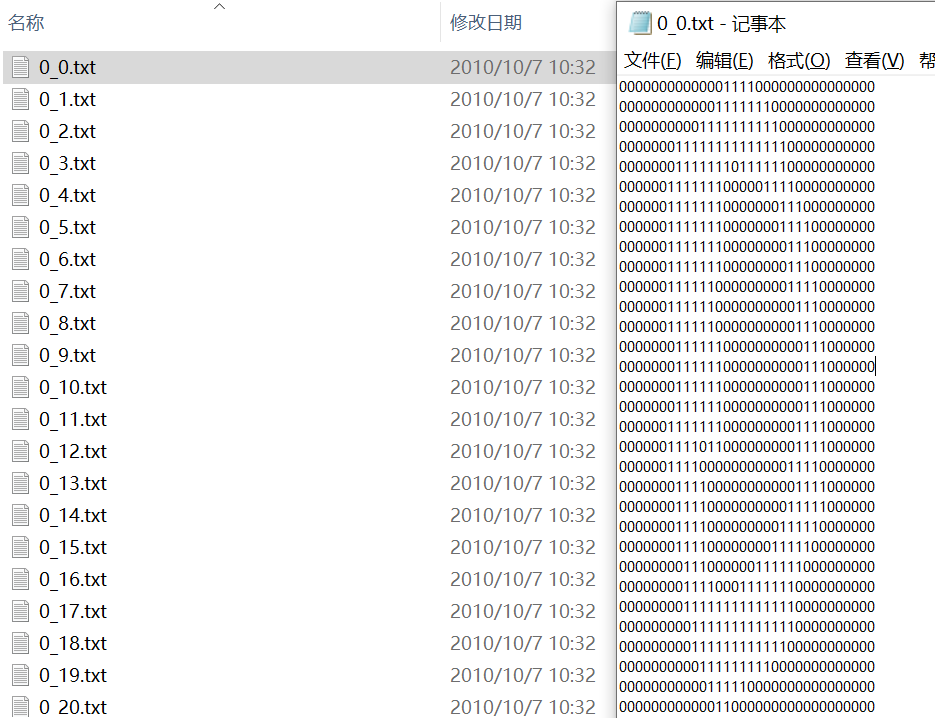# 函数img2vector将图像转换为向量
from numpy import *
from os import listdir

def img2vector(filename):
returnVect = np.zeros((1, 1024))
fr = open(filename)
for i in range(32):
for j in range(32):
returnVect[0, 32 * i + j] = int(lineStr[j])
return returnVect

# 读取手写字体txt数据
def handwritingData(dataPath):
hwLabels = []
FileList = listdir(dataPath)  # 1 获取目录内容
m = len(FileList)
np.Mat = np.zeros((m, 1024))
for i in range(m):
# 2 从文件名解析分类数字
fileNameStr = FileList[i]
fileStr = fileNameStr.split('.')  # take off .txt
classNumStr = int(fileStr.split('_'))
hwLabels.append(classNumStr)
np.Mat[i, :] = img2vector(dataPath + '/%s' % fileNameStr)
return np.Mat, hwLabels

数据集使用
将数据集解压到指定目录下，like this:
..
└ Work Folder
└ Net.py
trainingDigits
testDigits

# 读取训练数据
trainDataPath = "./trainingDigits"
trainMat, trainLabels = handwritingData(trainDataPath)

trainMat.shape -> (1934, 1024)
trainMat[i]对应的标签为trainLabels[i]

神经网络设计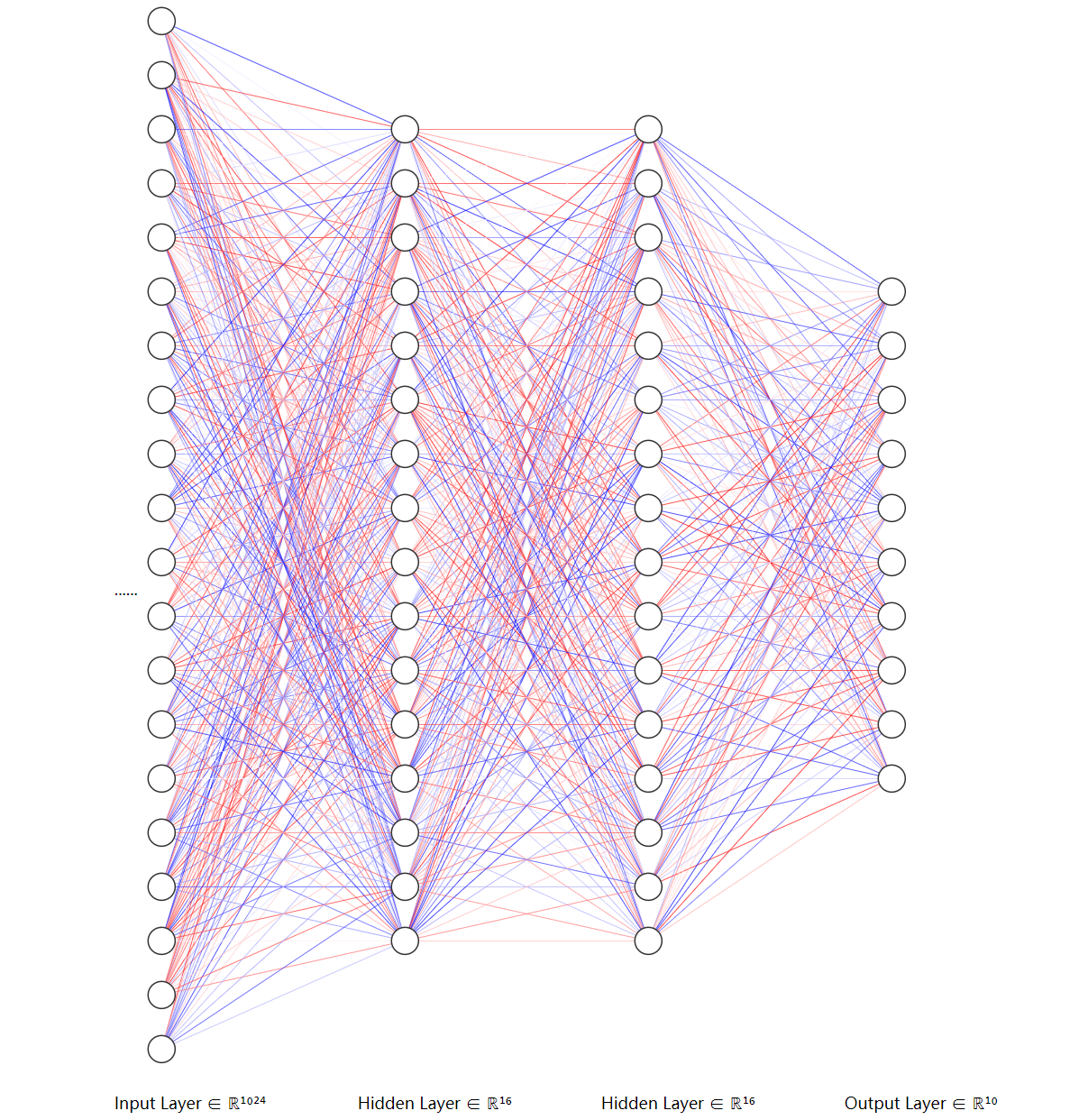这里是模型是这样的，如上图，一个输入层，两个隐含层，激活函数选择Sigmod,损失函数选择误差平方和函数（我这么称呼的，不知道叫什么），如下面所示。建议第一次自己些的话损失函数就用误差平方和函数，以为这个损失函数简单，求导方便，便于学习，后面可以在此基础上进行改进。
Cost ：代价，在这里也就是需要计算的Loss值。
$Sigmod(x) = \frac{1}{1+e^{-x}} \\ Sigmod'(x) = Sigmod(x)(1 - Sigmod(x)) \\$
$误差平方和函数: Cost( \hat{y} , y) = \frac{1}{2} ( \hat{y} - y)^2$
如果数学能力不够强的话建议不要用像交叉熵损失函数，也不要用Softmax激活函数这些求导麻烦的函数，不利于学习。
数学模型
$符号：A^{(层)}$
$S = Sigmod$

$维度： | \boldsymbol{x}_{1024x1} | \boldsymbol{W}_{16x1024}^{(1)} | \boldsymbol{b}_{16x1}^{(1)} | \boldsymbol{W}_{16x16}^{(2)} | \boldsymbol{b}_{16x1}^{(2)} | \boldsymbol{W}_{10x16}^{(3)} | \boldsymbol{b}_{10x1}^{(3)} | \boldsymbol{\hat{y}_{10x1}} |$

$前向传播：$
$\mathbf{z}^{(l)}=\mathbf{W}^{(l)} \mathbf{a}^{(l-1)}+\mathbf{b}^{(l)}$
$\mathbf{a}^{(l)}=f\left(\mathbf{z}^{(l)}\right)$
$\hat{\mathbf{y}}=\mathbf{a}^{(L)}=f\left(\mathbf{z}^{(L)}\right)$
$\mathcal{L}=\mathcal{L}(\mathbf{y}, \hat{\mathbf{y}})$

$\boldsymbol{x} = a^{(0)} 也就是输入的图片数据$
$\boldsymbol{z}^{(1)} = \boldsymbol{W}^{(1)}\boldsymbol{a}^{(0)}+\boldsymbol{b}^{(1)}$
$\boldsymbol{a}^{(1)} = S(\boldsymbol{z}^{(1)})$
$\boldsymbol{z}^{(2)} = \boldsymbol{W}^{(2)}\boldsymbol{a}^{(1)}+\boldsymbol{b}^{(2)}$
$\boldsymbol{a}^{(2)} = S(\boldsymbol{z}^{(2)})$
$\boldsymbol{z}^{(3)} = \boldsymbol{W}^{(2)}\boldsymbol{a}^{(2)}+\boldsymbol{b}^{(3)}$
$\boldsymbol{\hat{y}} = S(\boldsymbol{z}^{(3)}) 预测结果，最大值下标就是预测的数字结果$
$Loss = Cost( \boldsymbol{\hat{y}} , \boldsymbol{y})$

$反向传播:\odot 是对应元素相乘$
$\frac{\partial Loss}{\partial \boldsymbol{\hat{y}}}$:这里是对应元素求导
输出层：
$\boldsymbol{\delta}^{(L)}=\nabla_{\mathbf{a}^{(L)}} \mathcal{L} \odot S^{\prime}\left(\mathbf{z}^{(L)}\right) = \frac{\partial Loss}{\partial \boldsymbol{\hat{y}}} \odot S^{\prime}\left(\mathbf{z}^{(L)}\right) = \frac{\partial \mathcal{L} }{\partial \mathbf{z}^{(L)}}\\ 这里求得： \frac{\partial Loss}{\partial \boldsymbol{\hat{y}}} \odot S^{\prime}\left(\mathbf{z}^{(3)}\right)$
其他层套用公式：
$\boldsymbol{\delta}^{(l)}=\left(\left(\mathbf{W}^{(l+1)}\right)^{\mathrm{T}} \boldsymbol{\delta}^{(l+1)}\right) \odot S^{\prime}\left(\mathbf{z}^{(l)}\right) \\ \frac{\partial \mathcal{L}} {\partial\mathbf{W}^{(l)}}=\boldsymbol{\delta}^{(l)}\left(\mathbf{a}^{(l-1)}\right)^{\mathrm{T}} \\ \frac{\partial \mathcal{L}}{\partial \mathbf{b}^{(l)}}=\boldsymbol{\delta}^{(l)}$

$参数更新:$
\begin{aligned} \mathbf{W}^{(l)} &=\mathbf{W}^{(l)}-\alpha \frac{\partial \mathcal{L}}{\partial \mathbf{W}^{(l)}} \\ \mathbf{b}^{(l)} &=\mathbf{b}^{(l)}-\alpha \frac{\partial \mathcal{L}}{\partial \mathbf{b}^{(l)}} \end{aligned}
关于矩阵求导
如果你只是想能够自己求出反向传播过程中的梯度，那么记住上面的公式足够了，如果想要了解更多的有关公式可以参考这篇文章：神经网络求导。
标量$f$对矩阵$\boldsymbol{X}$的导数，定义为$\frac{\partial f}{\partial X}=\left[\frac{\partial f}{\partial X_{i j}}\right]$。
当然，你可能不想止步于此，可能对于一部分同学并没有接触过矩阵求导然后在反向传播公式推导的时候卡住了。想要了解如何进行矩阵求导可以参考：矩阵求导术。当然其实有个简单的办法，就是把矩阵的每个元素求解的等式连同它的下标写出来，像这样：$y_{i \times1} = \sum_{j} W_{i \times j} x_{j \times 1}$。然后再表示出偏导数，像这样：$\frac{\partial y_{i \times1}}{\partial W_{i \times j} } = x_{j \times 1}$。相当于只有$\mathbf{W}$的第$i$行$\boldsymbol{W}_{i \times \boldsymbol{j}}$做出了贡献，而$\mathbf{W}$的第$i$行$\boldsymbol{W}_{i \times \boldsymbol{j}}$(j相当于有个向量)也只对$y_{i \times1}$做贡献。
令$y_{i \times1} = \boldsymbol{W}_{i \times \boldsymbol{j}}\boldsymbol{x}_{\boldsymbol{j} \times 1} + \boldsymbol{b}_{i \times 1},$把$\boldsymbol{W}_{i \times \boldsymbol{j}}的每个元素W_{i \times j}$看作一个变量，$y_{i \times1}$相当于一个多元函数，元素$W_{i \times j}$增加1对$y_{i \times1}$的影响为$\frac{\Delta y_{i \times1}}{\Delta W_{i \times j} }$,取极限为$\frac{\partial y_{i \times1}}{\partial W_{i \times j} } = x_{j \times 1}$， 由于是只有第$\boldsymbol{W}$的第$i$列，有影响，所以最后求出来的梯度应该是一行，这行的值转置就是$\boldsymbol{x}$。$\boldsymbol{x}$求导时看作常数。

说人话，如下图： 假设$i = 1$。
$\frac{\partial {y}_{i \times1}}{\partial \boldsymbol{W}_{i \times \boldsymbol{j}} } = \left[ \begin{matrix} \frac{\partial y_{1 \times1}}{\partial W_{1 \times 1} } =x_{1 \times 1} & \frac{\partial y_{1 \times1}}{\partial W_{1 \times 2} } =x_{2 \times 1} & \cdots & \frac{\partial y_{i \times1}}{\partial W_{i \times j} } = x_{j \times 1} \\ \end{matrix} \right] \\ =\left[ \begin{matrix} x_{1 \times 1} \\ x_{2 \times 1} \\ \vdots \\ x_{j \times 1} \\ \end{matrix} \right]^T = \boldsymbol{x}^T$
当然，这个证明不完整，我觉得剩下的不需要(懒得 )写了。注意这是变量对矩阵的导数，虽然$\boldsymbol{y}$有多项，但是分别来求的。又因为$Loss$是是把$\boldsymbol{y}$求和的函数，所以根据链式求导法则：
$\boldsymbol{y} = \boldsymbol{W} \boldsymbol{x} \\ Loss = C(\boldsymbol{y}) \\ \frac{\partial Loss }{\partial \boldsymbol{W} } = \frac{\partial Loss }{\partial \boldsymbol{y} } \frac{\partial \boldsymbol{y}}{\partial \boldsymbol{W} }$
$\frac{\partial Loss }{\partial \boldsymbol{y} }$是逐项求导，得到向量$\boldsymbol{\delta}$正好把每个$\frac{\partial y_{i \times1}}{\partial W_{i \times j} }$"搬到"对应行。
$\frac{\partial Loss }{\partial \boldsymbol{W} } = \frac{\partial Loss }{\partial \boldsymbol{y} } \boldsymbol{x}^T$

完整解析：
$\frac{\partial Loss }{\partial \boldsymbol{W} } = \frac{\partial Loss }{\partial \boldsymbol{y} } \frac{\partial \boldsymbol{y}}{\partial \boldsymbol{W} } = \\ \left[ \begin{matrix} \frac{\partial Loss }{\partial {y_{1 \times 1}} } \frac{\partial {y}_{1 \times1}}{\partial \boldsymbol{W}_{1 \times \boldsymbol{j}} }\\ \frac{\partial Loss }{\partial {y_{2 \times 1}} } \frac{\partial {y}_{2 \times1}}{\partial \boldsymbol{W}_{2 \times \boldsymbol{j}} } \\ \vdots \\ \frac{\partial Loss }{\partial {y_{i \times 1}} } \frac{\partial {y}_{i \times1}}{\partial \boldsymbol{W}_{i \times \boldsymbol{j}} } \\ \end{matrix} \right] = \left[ \begin{matrix} \frac{\partial Loss }{\partial {y_{1 \times 1}} } \frac{\partial {y}_{1 \times1}}{\partial {W}_{1 \times {1}} } = x_{1 \times 1} & \frac{\partial Loss }{\partial {y_{1 \times 1}} } \frac{\partial {y}_{1 \times1}}{\partial {W}_{1 \times {2}} } = x_{2 \times 1} & \cdots & \frac{\partial Loss }{\partial {y_{1 \times 1}} } \frac{\partial {y}_{1 \times1}}{\partial {W}_{1 \times {j}} } = x_{j \times 1} \\ \frac{\partial Loss }{\partial {y_{2 \times 1}} } \frac{\partial {y}_{2 \times1}}{\partial {W}_{2 \times {1}} } = x_{1 \times 1} & \frac{\partial Loss }{\partial {y_{2 \times 1}} } \frac{\partial {y}_{2 \times1}}{\partial {W}_{2 \times {2}} } = x_{2 \times 1} & \cdots & \frac{\partial Loss }{\partial {y_{2 \times 1}} } \frac{\partial {y}_{2 \times1}}{\partial {W}_{2 \times {j}} } = x_{j \times 1} \\ \vdots & \vdots & \ddots & \vdots \\ \frac{\partial Loss }{\partial {y_{i \times 1}} } \frac{\partial {y}_{i \times1}}{\partial {W}_{i \times {1}} } = x_{1 \times 1} & \frac{\partial Loss }{\partial {y_{i \times 1}} } \frac{\partial {y}_{i \times1}}{\partial {W}_{i \times {2}} } = x_{2 \times 1} & \cdots & \frac{\partial Loss }{\partial {y_{i \times 1}} } \frac{\partial {y}_{i \times1}}{\partial {W}_{i \times {j}} } = x_{j \times 1} \\ \end{matrix} \right] \\ =\frac{\partial Loss }{\partial \boldsymbol{W} } = \frac{\partial Loss }{\partial \boldsymbol{y} } \frac{\partial \boldsymbol{y}}{\partial \boldsymbol{W} } = \\ \left[ \begin{matrix} \frac{\partial Loss }{\partial {y_{1 \times 1}} } \\ \frac{\partial Loss }{\partial {y_{2 \times 1}} } \\ \vdots \\ \frac{\partial Loss }{\partial {y_{i \times 1}} } \\ \end{matrix} \right] \left[ \begin{matrix} x_{1 \times 1} &x_{2 \times 1} & \cdots &x_{j \times 1} \end{matrix} \right] = \frac{\partial Loss }{\partial \boldsymbol{y} } \boldsymbol{x}^T$
我没办法从数学上严格证明，但是，从上面的推导来看肯定是对的。当然推荐你看看矩阵求导术。其次上面的都是标量对矩阵或者向量的求导。
这样你大概能够看得出来导数长什么样。 我忘了怎么推导，但是我有个更好的主意，从梯度$\nabla$入手。
程序实现
如下，这里初始化选择可以是全1或者是全0，但是那样效果会不好，收敛速度非常慢（为什么？以后慢慢研究）。可以使用NumPy的randn()和rand()来进行初始化，numpy.random.rand(d0, d1, …, dn)的随机样本位于[0, 1)范围内，numpy.random.randn(d0, d1, …, dn)是按照标准正态分布生成随机值。当然两种初始化方式收敛速度和稳定性略有不同，稍后讨论。
这里给出神经网络的主要的代码，完整的项目见附录的下载地址。代码中的变量名字尽量按照前面的公式中的结果一一对应，便于理解。例如：y_hat指的就是神经网络的输出$\boldsymbol{\hat{y}}$，dW1指的是$\frac{\partial \mathcal{Loss}}{\partial \mathbf{W}^{(1)}}$, alpha指的是学习率$\alpha$,SquareErrorSum指的是代价函数$Cost( \boldsymbol{\hat{y}} , \boldsymbol{y})$,以此类推。
#!/usr/bin/python3
# coding:utf-8
# @Author: Lin Misaka
# @File: net.py
# @Data: 2020/11/30
# @IDE: PyCharm
import numpy as np
import matplotlib.pyplot as plt

# diff = True求导
def Sigmoid(x, diff=False):
def sigmoid(x):        # sigmoid函数
return 1 / (1 + np.exp(-x))
def dsigmoid(x):
f = sigmoid(x)
return f * (1 - f)
if (diff == True):
return dsigmoid(x)
return sigmoid(x)

# diff = True求导
def SquareErrorSum(y_hat, y, diff=False):
if (diff == True):
return y_hat - y
return (np.square(y_hat - y) * 0.5).sum()

class Net():
def __init__(self):
# X Input
self.X =  np.random.randn(1024, 1)
self.W1 = np.random.randn(16, 1024)
self.b1 = np.random.randn(16, 1)

self.W2 = np.random.randn(16, 16)
self.b2 = np.random.randn(16, 1)

self.W3 = np.random.randn(10, 16)
self.b3 = np.random.randn(10, 1)
self.alpha = 0.01  #学习率
self.losslist = [] #用于作图

def forward(self, X, y, activate):
self.X = X
self.z1 = np.dot(self.W1, self.X) + self.b1
self.a1 = activate(self.z1)
self.z2 = np.dot(self.W2, self.a1) + self.b2
self.a2 = activate(self.z2)
self.z3 = np.dot(self.W3, self.a2) + self.b3
self.y_hat = activate(self.z3)
Loss = SquareErrorSum(self.y_hat, y)
return Loss, self.y_hat

def backward(self, y, activate):
self.delta3 = activate(self.z3, True) * SquareErrorSum(self.y_hat, y, True)
self.delta2 = activate(self.z2, True) * (np.dot(self.W3.T, self.delta3))
self.delta1 = activate(self.z1, True) * (np.dot(self.W2.T, self.delta2))
dW3 = np.dot(self.delta3, self.a2.T)
dW2 = np.dot(self.delta2, self.a1.T)
dW1 = np.dot(self.delta1, self.X.T)
d3 = self.delta3
d2 = self.delta2
d1 = self.delta1
#update weight
self.W3 -= self.alpha * dW3
self.W2 -= self.alpha * dW2
self.W1 -= self.alpha * dW1
self.b3 -= self.alpha * d3
self.b2 -= self.alpha * d2
self.b1 -= self.alpha * d1

def setLearnrate(self, l):
self.alpha = l

def train(self, trainMat, trainLabels, Epoch=5, bitch=None):
for epoch in range(Epoch):
acc = 0.0
acc_cnt = 0
label = np.zeros((10, 1))#先生成一个10x1是向量，减少运算。用于生成one_hot格式的label
for i in range(len(trainMat)):#可以用batch，数据较少，一次训练所有数据集
X = trainMat[i, :].reshape((1024, 1)) #生成输入

labelidx = trainLabels[i]
label[labelidx] = 1.0

Loss, y_hat = self.forward(X, label, Sigmoid)#前向传播
self.backward(label, Sigmoid)#反向传播

label[labelidx] = 0.0#还原为0向量
acc_cnt += int(trainLabels[i] == np.argmax(y_hat))

acc = acc_cnt / len(trainMat)
self.losslist.append(Loss)
print("epoch:%d,loss:%02f,accrucy : %02f" % (epoch, Loss, acc))
self.plotLosslist(self.losslist, "Loss")

运行结果
学习率为0.01，迭代全部数据1743次的时候准确率达到了98.7%。下面是几条记录：
epoch:0,loss:0.483388,accrucy : 0.107032
epoch:1,loss:0.443391,accrucy : 0.217684
epoch:16,loss:0.415309,accrucy : 0.453981
epoch:88,loss:0.454633,accrucy : 0.834540
epoch:137,loss:0.491693,accrucy : 0.901758
epoch:810,loss:0.045566,accrucy : 0.977249
epoch:1420,loss:0.009614,accrucy : 0.985522
epoch:1743,loss:0.008058,accrucy : 0.987073

Loss图：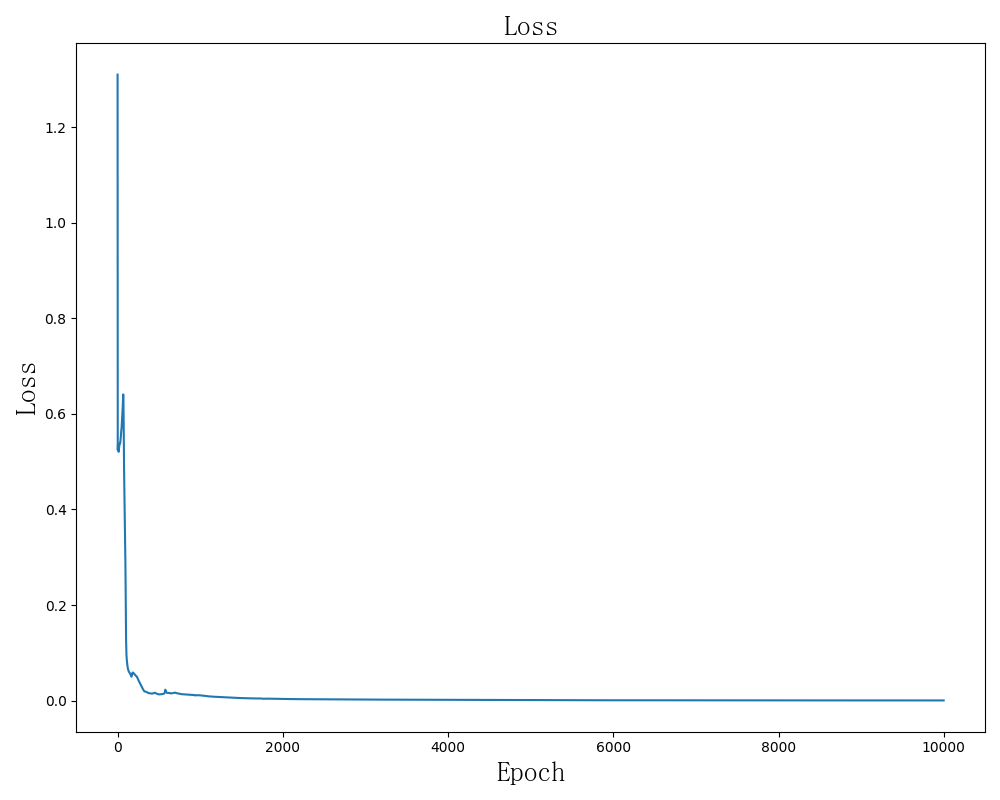关于权值初始化
不同初始化方法收敛速度分析：
学习率为0.01。修改初始化的地方在这里：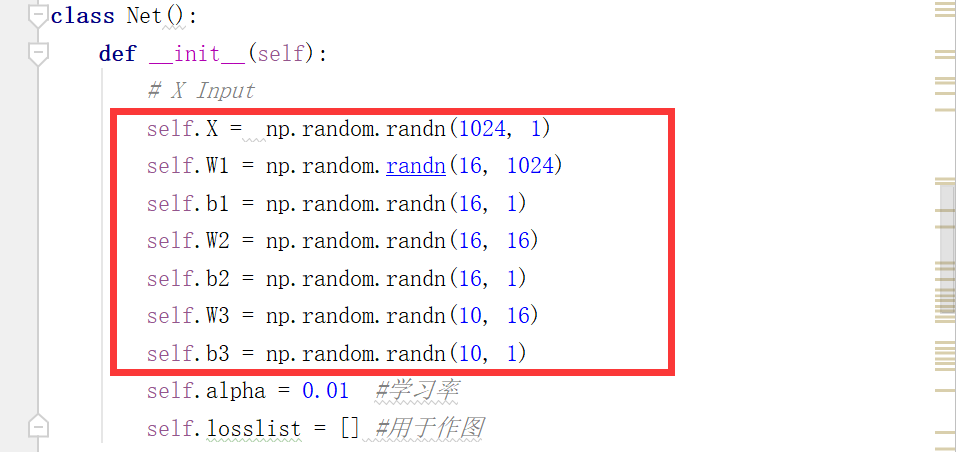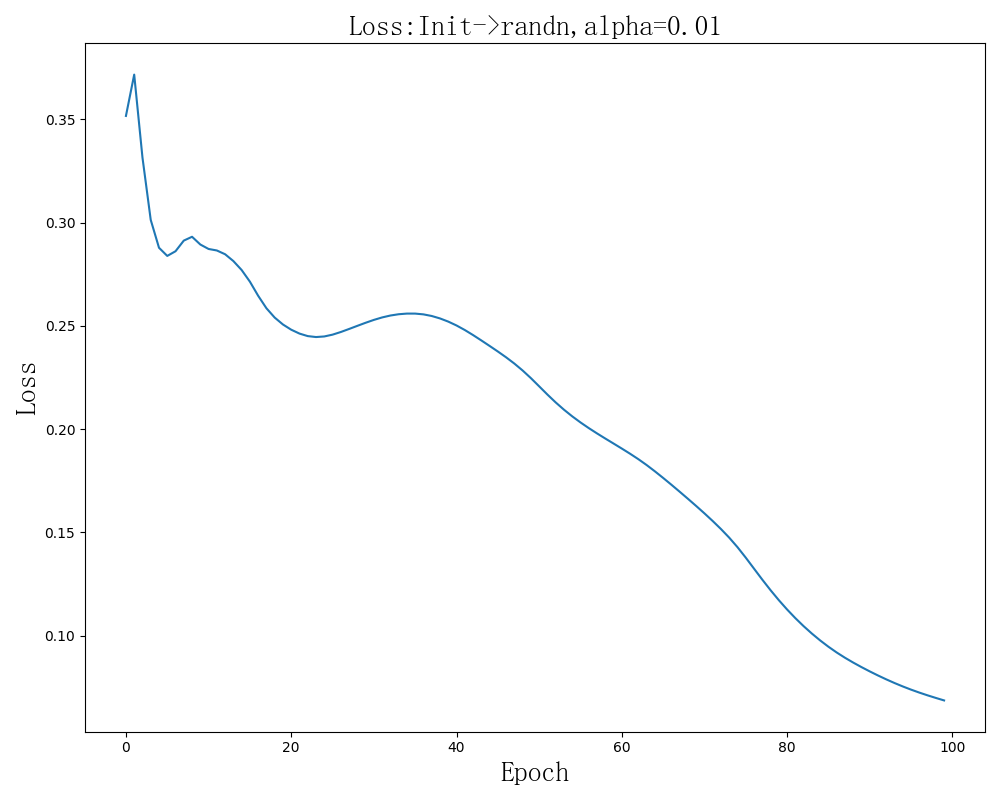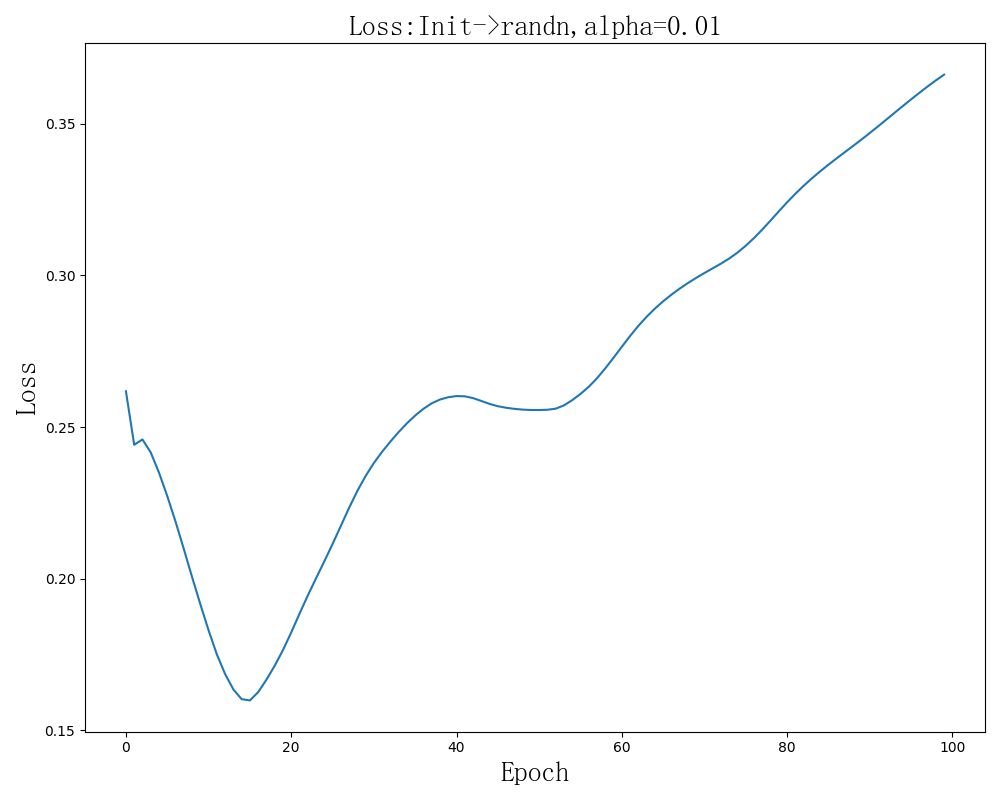似乎有时候会出现Loss增大的情况，但是随机的，与初始化值和学习率有关。
第一幅图最终结果：epoch:99,loss:0.068638,accrucy : 0.903309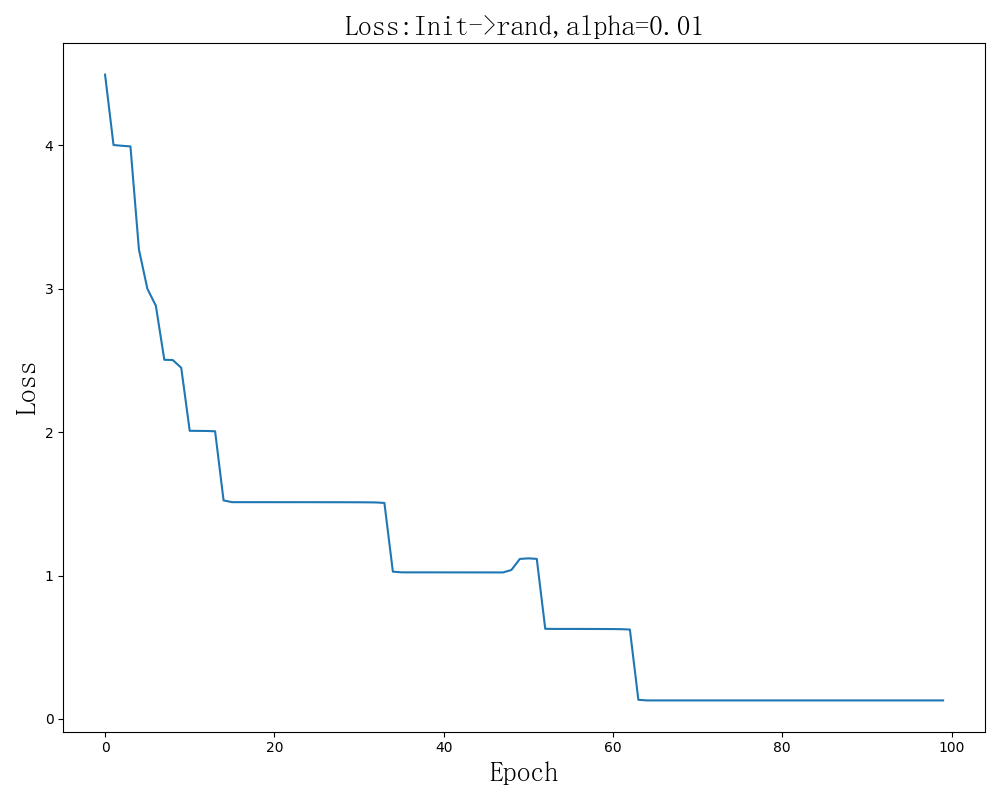效果很差。
epoch:99,loss:0.129023,accrucy : 0.307653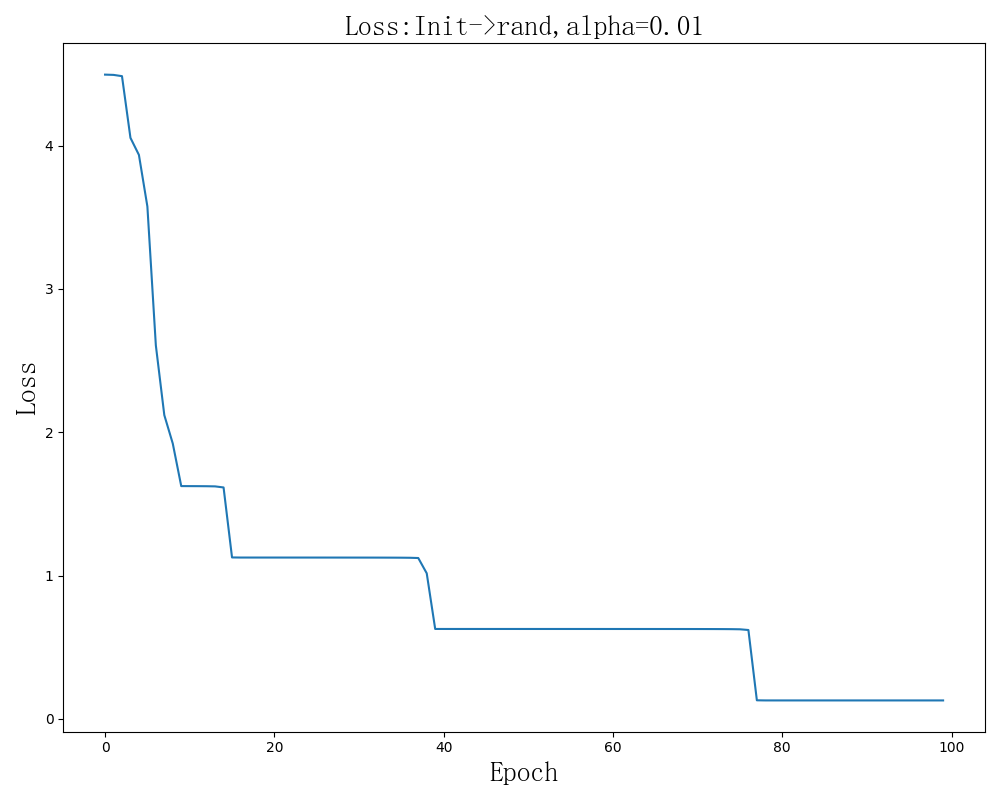效果有一次很差，我想大胆推断这样初始化的效果都很差，但是这样不科学，我试着改变学习率为0.1，效果有所提升。
epoch:99,loss:0.129075,accrucy : 0.307653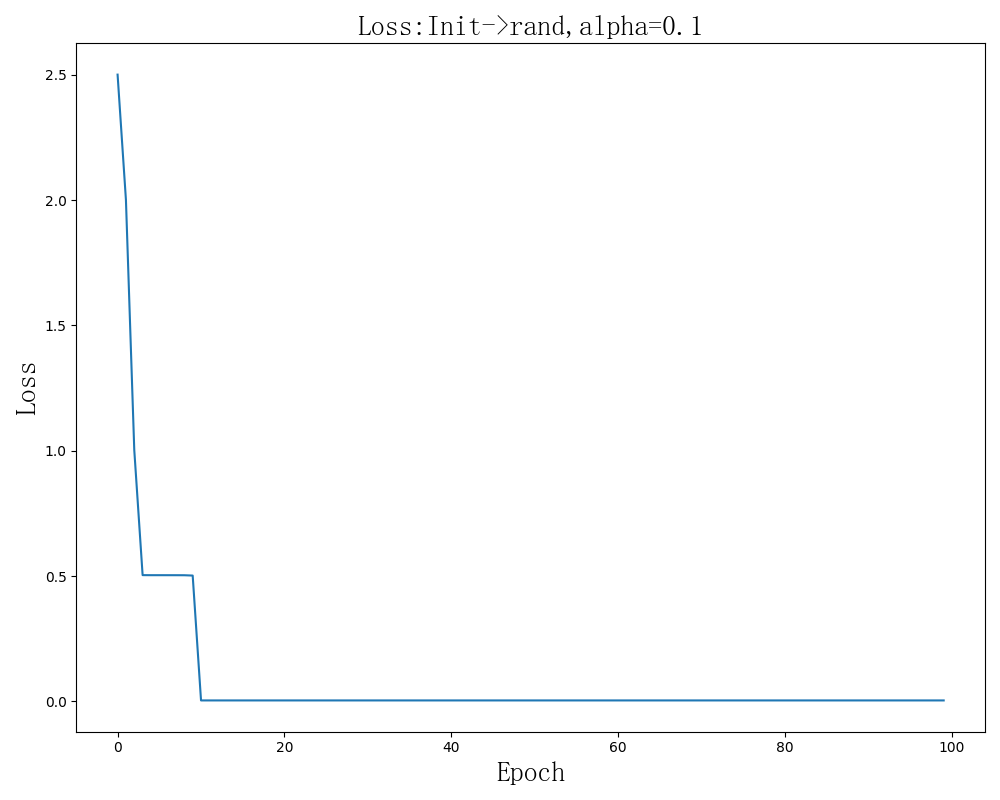epoch:99,loss:0.003670,accrucy : 0.767322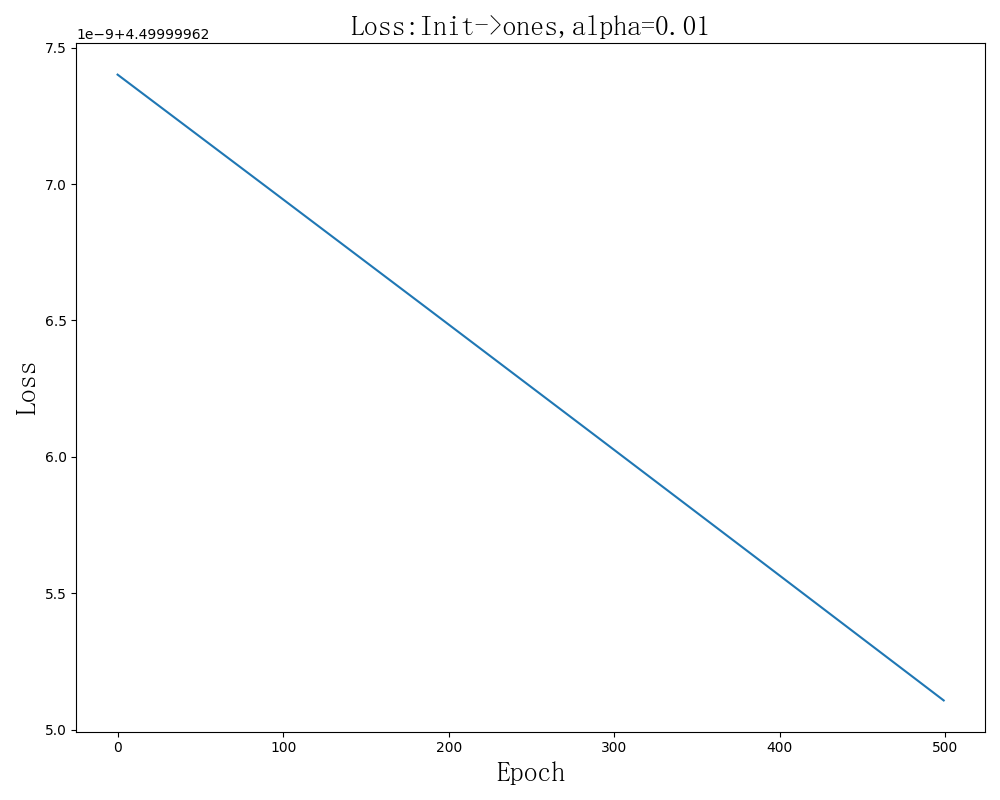这种初始化方式可见非常糟糕，虽然几乎是线性下降，但是下降速度简直不要太慢。
epoch:0,loss:4.500000,accrucy : 0.103413
epoch:12,loss:4.500000,accrucy : 0.103413
epoch:13,loss:4.500000,accrucy : 0.103930
epoch:499,loss:4.500000,accrucy : 0.105481

注： 偏置b初始化的方式对收敛影响不大，这个读者可以自己试试。
为什么不同的初始化收敛速度不一样？
待续~
关于损失函数
待续~
关于我的感想
隐含层神经元数量不是越多越好，我以前用PyTroch试过训练和这个网络差不多的有个网络，输入层，输出层和这个神经网络一样，开始隐含层有64和24个神经元，效果含不错，但是我试着改变隐含层神经元的数量使之更多，效果反而不好，并且由于运算量增加，迭代速度大大下降。
从上面的实验结果看似乎权值初始化的时候使用标准正态分布的随机值会比较好，但这是为什么还值得继续学习。此外似乎偏置b初始化的方式对收敛影响不大，这个读者可以自己试试。

关于学习神经网络，如果你是以实用主义来思考的，那么可能你就只需把机器学习领域的算法了解一遍，学会用框架就可以了。但是这也就只是会用罢了。
倘如你想要研究这些网络，试图提高它的准确性、收敛速度、预测速度、内存占用、复杂度等等各种性能，以至于创造一种全新的结构、全能的结构、可以组合的结构等等都需要对它们有深入的了解，而不是只会使用框架这种皮毛。其次学习过程也不能囫囵吞枣，要循序渐进，以实践总结理解为主。

关于欺骗神经网络，神经网络漏洞
附录
文章资源
手写数字识别全连接神经网络教学Demo：将digits.zip解压到net.py，安装好环境即可运行。
git clone https://github.com/MisakaMikoto128/FCNNeuralNetworkDemoForHandwrittenDigits.git

备份。
参考
 神经网络求导
 常见的损失函数(loss function)总结
 矩阵求导术（上）
 矩阵求导术（下）
 小白都能看懂的softmax详解
链接失效Call我。
github:https://github.com/MisakaMikoto128
个人网站


展开全文机器学习 深度学习 神经网络 NumPy...